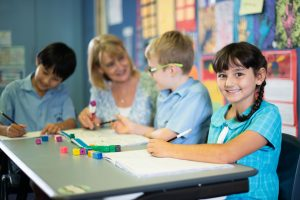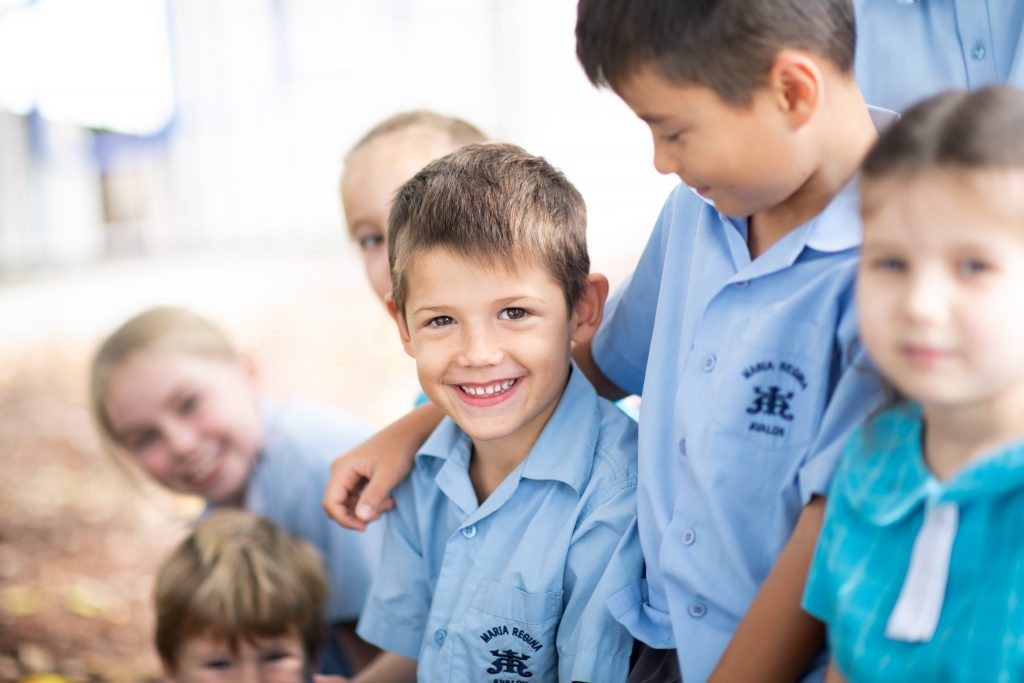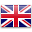Learning

# Mathematics

Mathematics is the Key Learning Area (KLA) where students appreciate mathematics as an essential and relevant part of life and develop their ability to work mathematically.Mathematics provides students with essential mathematical skills and knowledge in Number and Algebra, Measurement and Geometry and Statistics and Probability. Tasks in Mathematics are designed to spark curiosity in learners and cater for a wide range of student abilities. The focus is on building student confidence in using increasingly sophisticated understandings and strategies to respond to Mathematical problems. Learners are challenged to make informed decisions and to interpret and apply mathematics in a variety of contexts in preparation for life in the 21st century.

Growth Mindset is nurtured in all students throughout the school. The aim is to produce Mathematicians who are inspired to ask questions, apply their understanding creatively, take risks in their learning and relate their understanding to the world around them. Technology is an integral part of the curriculum and a wide range of programmes and Apps are used to enhance and enrich Mathematical understandings.Student growth in the development of number concepts is tracked at the beginning of the school year from Kindergarten through to Year 6. This data is used to develop each student’s strategies and confidence in whole number, place value, addition and subtraction and multiplication and division.

The EMU programme (Extending Mathematical Understandings) is run at Maria Regina to assist vulnerable students in the early years. Classroom teachers also target vulnerable students in their class group through a wide range of Mathematics games and activities

NESA Mathematics SyllabusEnglish